Announcements
Applications are open for the 2024 Qlik Luminary Program. Apply by December 15 here.
cancel
Showing results for
Did you mean: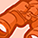Contributor III

## Sum of aggregate by dimension

This is my source data:

Order IDValue AValue B
A26
B54
B52
C15
C15
C14
D68

I want my result table in Qlik Sense to be:

Order IDDistinct Sum Value ASUM Value B
A26
B56
C114
D68
Total1434

I tried everything with Aggr, Distinct and Sum, but haven't succeeded yet. Who can point me in the right direction? I would be very grateful!

11 RepliesEmployee

Hi,

It looks like you have done a left join in the script on OrderID and because there are multiple values of B for each orderID, A values have been duplicated. It would be best not to join the two levels and let Qlik show the correct sum.

Alternately, if you have not joined tables in the script, I would take care of this in the script editor and create two tables from this single table. Table1: Should have OrderID and FirstValue(ValueA) and group by OrderID. Table 2: As is table without ValueA. Now the frontend table would show as desiredMVP

Try this for Value A

Sum(Aggr(Only([Value A]), [Order ID]))

or

Sum(Aggr(Min([Value A]), [Order ID]))

or

Sum(Aggr(Max([Value A]), [Order ID]))

or

Sum(Aggr(Avg([Value A]), [Order ID]))

or

Sum(Aggr(Sum(DISTINCT [Value A]), [Order ID]))

For Value B just Sum([Value B]) should workMVP

Here you are (attached a sample)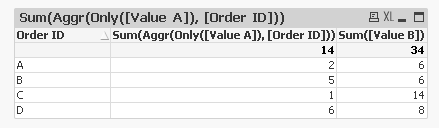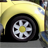Specialist

Dimension: Order ID

Equation:

A: Sum(DISTINCT [Value A])

B: Sum([Value B])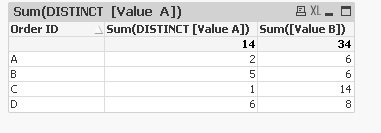Contributor III
Author

Thanks, but the problem happens when I introduce another dimension, it will show 0 for the next rows, while I want it to show the Value of A. I only want it to affect the total.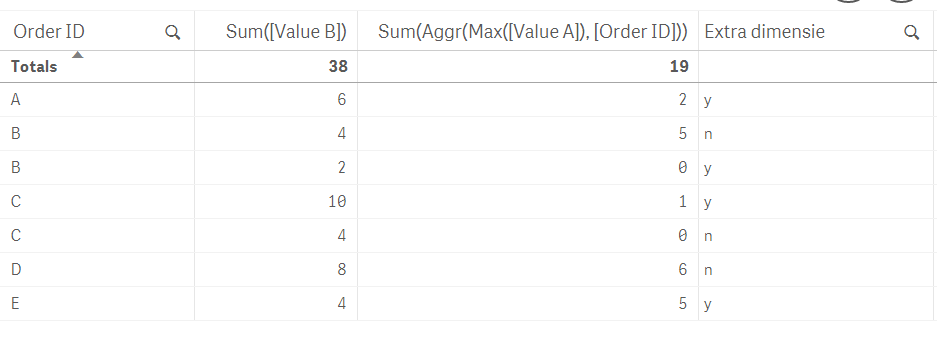MVP

Not sure what you are expecting to see, but may be you need to add your second dimension to the Aggr() functionSpecialist IIContributor III
Author

I want 19 as a total result in the example above, but I want it to show it's value of A on each line. When I add the Extra dimension to the calculation, the total gets distorted..
I am afraid it's not possible what I want with the aggr. formula. Splitting up the tables gives the correct result, but it has implications for the rest of my datamodel. (another story).Contributor III
Author

Yeah unfortunately I have to join, because of other implications in my data model. Might need to do some concessions though and keep them seperated.Tags
Community Browser